# Model and Analyze Dual Polarized Patch Microstrip Antenna

This example shows how to design and measure a wideband dual polarized microstrip antenna that finds its use at the base station of a cellular system. In order to achieve the wideband characteristics, this design considers a slot coupled patch antenna structure.

### Building Aperture Coupled Antenna

#### Define Parameters

Parameters given below define the offsets for upper and lower slots and the stublengths.

```of_1 = 12e-3; of_2 = -6e-3; LM = 7e-3; LM_2 = 7e-3;```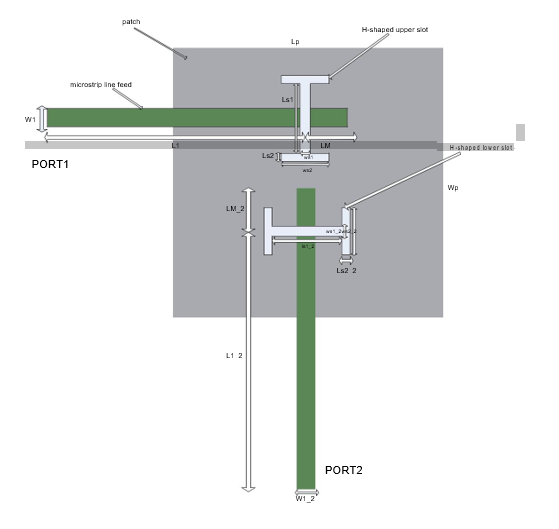Refer  pp. 55

### Define Patch

In this antenna, the radiating element is patchMicrostrip. Above the patch, a dielectric substrate with EpsilonR of 3.38, acts like a radome. Below the patch there is a foam material of EpsilonR 1.025.

```Lp = 50e-3; patch = antenna.Rectangle(Length=Lp,Width=Lp,Center=[0 0]);```

### Define H-shaped Slots

The double slots perform dual polarized operation. Each slot is H-shaped and positioned on the ground plane in "T" formation. This formation provides a good isolation level between port1 and port2.

Define upper H-shape Slot

```Ls1 = 12e-3; Ws1 = 0.5e-3; Ls2 = 1e-3; Ws2 = 22e-3; f1 = antenna.Rectangle(Length=Ws1,Width=Ls1,Center=[0 of_1]); f2 = antenna.Rectangle(Length=Ws2,Width=Ls2,Center=[0 of_1+(Ls1/2)+(Ls2/2)]); f3 = antenna.Rectangle(Length=Ws2,Width=Ls2,Center=[0 of_1-(Ls1/2)-(Ls2/2)]); f4 = f1 + f2 + f3;```

Define lower H-shape Slot

```Ls1_2 = 17e-3; Ls2_2 = 1e-3; Ws1_2 = 0.5e-3; Ws2_2 = 17e-3; f5 = antenna.Rectangle(Length=Ls1_2, Width=Ws1_2, Center=[0 of_2]); f6 = antenna.Rectangle(Length=Ls2_2, Width=Ws2_2, Center=[(Ls1_2/2)+(Ls2_2/2) of_2]); f7 = antenna.Rectangle(Length=Ls2_2, Width=Ws2_2, Center=[-((Ls1_2/2)+(Ls2_2/2)) of_2]); f8 = f5 + f6 + f7;```

### Define Ground Plane

Create the ground plane shape for the antenna. The ground plane in this case is a square of size 100mm x 100mm.

```LGp = 100e-3; Ground_plane = antenna.Rectangle(Length=LGp, Width=LGp, Center=[0 0]);```

### Define Slotted Ground Plane

Use the rectangle shape primitives to create the H-slots. Use the Boolean subtraction operation to slot the Ground plane.

```Gp_slot = Ground_plane - f4 - f8; figure show(Gp_slot);```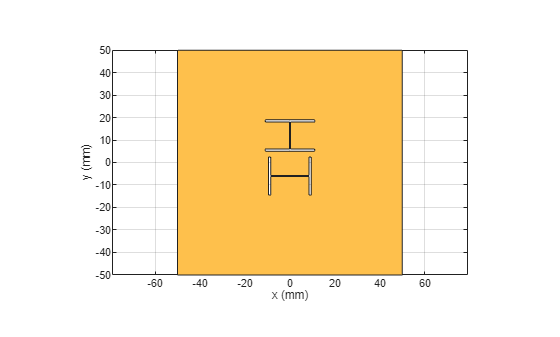### Define Feed Lines

Use a feed line of size 50mm x 1.181mm for the upper H-slot. Use a feed line of size 44mm x 1.181mm for the lower H-slot. Connect the stubs at the end of the feed lines.

```L1 = 50e-3; W1 = 1.181e-3; L1_2 = 44e-3; W1_2 = 1.181e-3; feed_1 = antenna.Rectangle(Length=L1,Width=W1,Center=[-(L1/2) of_1]); feed_2 = antenna.Rectangle(Length=W1_2,Width=L1_2,Center=[0 -((L1_2/2))+(of_2)]); feed_1_2 = feed_1 + feed_2; stub_1 = antenna.Rectangle(Length=LM,Width=W1,Center=[(LM/2) of_1]); stub_2 = antenna.Rectangle(Length=W1,Width=LM_2,Center=[0 of_2/2]); stub = stub_1 + stub_2; feed = feed_1_2 + stub; figure show(feed);```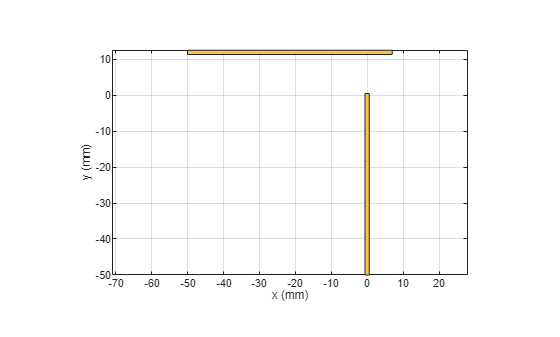### Define PCB Stack

Use the pcbStack to define the metal and dielectric layers and the feed for the aperture coupled patch antenna. The layers are defined top-down. In this case, the top-most layer is a dielectric layer. The second layer is a patch of square shape, and the third layer is another dielectric, followed by a fourth layer which is the ground plane. Fifth Layer is again the same dielectric which is used as first layer. Sixth layer is related to feed lines. Consider only the layers below the metal patch to calculate the board thickness as the layers above the metal patch are considered as coating and not included in the board thickness.

```p = pcbStack; d1 = dielectric(EpsilonR=3.38,Thickness=0.51e-3,Name="RO4003"); d2 = dielectric(EpsilonR=1.025,Thickness=14e-3,Name="Foam"); p.BoardThickness = d1.Thickness + d2.Thickness```
```p = pcbStack with properties: Name: 'MyPCB' Revision: 'v1.0' BoardShape: [1x1 antenna.Rectangle] BoardThickness: 0.0145 Layers: {[1x1 antenna.Rectangle] [1x1 antenna.Rectangle]} FeedLocations: [-0.0187 0 1 2] FeedDiameter: 1.0000e-03 ViaLocations: [] ViaDiameter: [] FeedViaModel: 'strip' FeedVoltage: 1 FeedPhase: 0 Conductor: [1x1 metal] Tilt: 0 TiltAxis: [1 0 0] Load: [1x1 lumpedElement] ```
```p.BoardShape.Length = LGp; p.BoardShape.Width = LGp; p.Layers = {d1,patch,d2,Gp_slot,d1,feed}; p.FeedLocations = [-L1 of_1 4 6;0 -L1_2+of_2 4 6]; p.FeedDiameter=feed_1.Width/3; figure show(p);```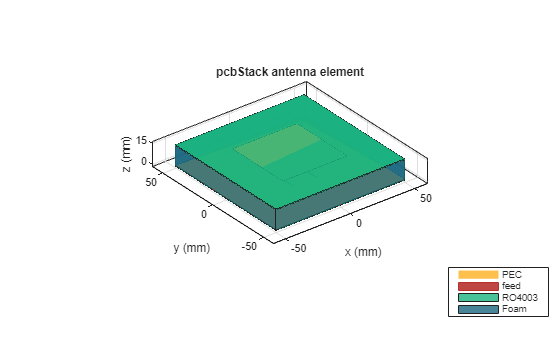Plot the radiation pattern of the antenna at the frequencies of best match. Use a resonant frequency of 1.79 GHz to plot the radiation pattern.

```figure pattern(p,1.79e9);```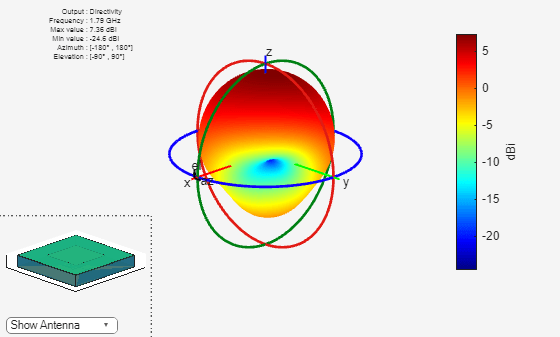### Meshing Antenna

Mesh the antenna with maximum edge length of 0.036m.

``` figure mesh(p,MaxEdgeLength=0.036);```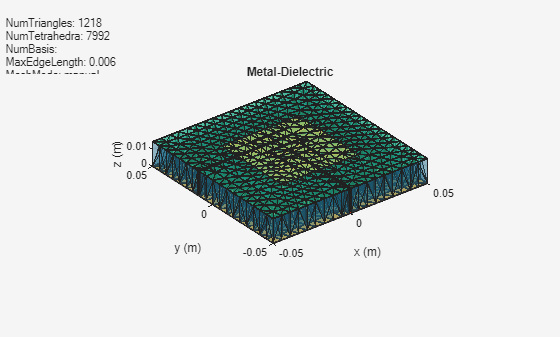### Calculate and Plot S-parameters

The plot shows return loss characteristics(S11,S22) and isolation(S12) between ports.

```figure sf = sparameters(p,linspace(1.6e9,2e9,20)); rfplot(sf);```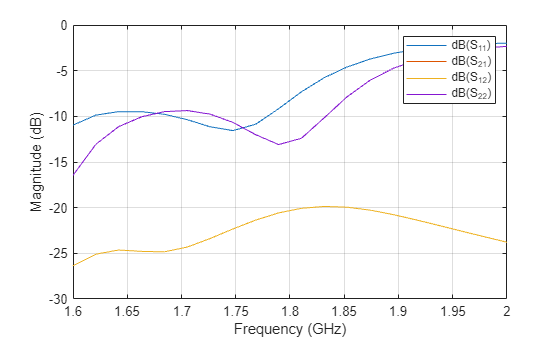### Plot Impedance Pattern

Use a frequency range from 1.5 GHz to 1.9 GHz with 20 frequency points to plot the impedance pattern.

```figure impedance(p,linspace(1.5e9,1.9e9,20),1);```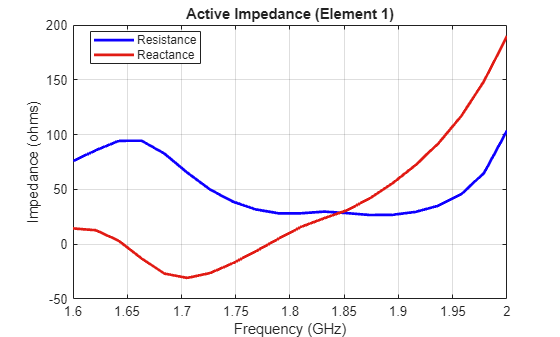```figure impedance(p,linspace(1.5e9,1.9e9,20),2);```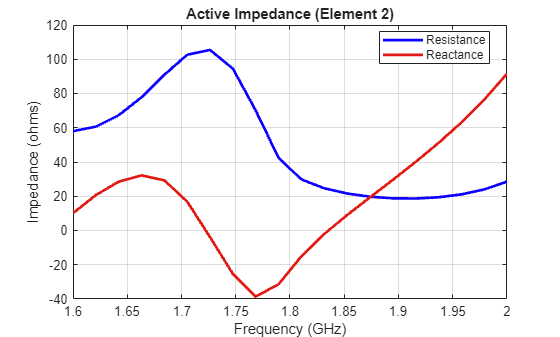### Conclusion

The design and analysis of the dual polarized aperture coupled antenna using Antenna Toolbox agrees well with the referred results.

### References

 Meltem Yildirim, "Design of Dual Polarized Wideband Microstrip Antennas", pp. 54-70.